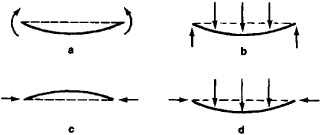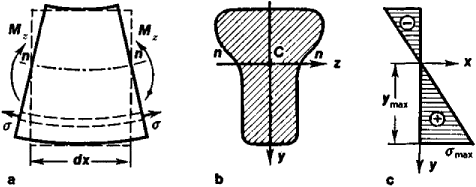Bending

(redirected from bendingly)
Also found in: Dictionary, Thesaurus.

bending

[′ben·diŋ]
(engineering)
The forming of a metal part, by pressure, into a curved or angular shape, or the stretching or flanging of it along a curved path.
The forming of a wooden member to a desired shape by pressure after it has been softened or plasticized by heat and moisture.
(hydrology)
Movement of sea ice up or down resulting from lateral pressure exerted by wind or tide.
(cell and molecular biology)
A conformational change characterized by a localized bend or kink in deoxyribonucleic acid due to heterogeneities in local structural composition.
(oceanography)
The first stage in the formation of pressure ice caused by the action of current, wind, tide, or air temperature changes.
McGraw-Hill Dictionary of Scientific & Technical Terms, 6E, Copyright © 2003 by The McGraw-Hill Companies, Inc.
The following article is from The Great Soviet Encyclopedia (1979). It might be outdated or ideologically biased.

Bending

an operation in forging, hot stamping, and cold stamping in which all or part of a workpiece is bent into a curved shape. Bending also refers to the mechanical operation of curving blanks from shaped materials. The bending moment produced by the process deforms the workpiece, stretching its outer layers and compressing its inner layers. Bending is done on bulldozers, roller and rotary bending machines (sheet-bending and section-bending machines), machines for bending with tension, and so on. Bending and tension machines are widely used because springing and crimping of the workpiece are eliminated. Machine parts, instruments, and a variety of metal goods are produced by bending.

REFERENCE

Storozhev, M. V., and E. A. Popov. Teoriia obrabotki metallov davleniem, 2nd ed. Moscow, 1963.

D. I. BRASLAVSKII

Bending

(in strength of materials) a type of deformation characterized by twisting (a change in curvature) of the axis or middle surface of the object being deformed (such as a beam, girder, plate, or shell) under the action of external forces or of temperature. A distinction is made between simple, or plane, bending, in which the external forces lie in a principal plane of the beam (that is, a plane passing through its axis and the main axes of inertia of the cross section); complex bending, which is caused by forces lying in different planes; and unsymmetrical bending, which is a particular case of complex bending. Depending on the force factors acting in the cross section of the beam (Figure 1, a and b), bending may be pure (in the presence of only bending moments) or transverse (when transverse forces are also present). A special case of bending—buckling (Figure l,c), which is characterized by swelling of a rod under the action of longitudinal compressive forces—is considered in engineering practice. The simultaneous action of forces directed along the axis of the rod and perpendicular to it causes transverse buckling (Figure l, d).Figure 1. Bending of a beam: (a) pure bending, (b) transverse bending, (c) buckling, (d) transverse buckling

The approximate design of a straight beam for the action of bending in the elastic stage is made on the assumption that the cross sections of the beam are flat until bending and remain flat even after bending (the hypothesis of flat sections); it is also assumed that upon bending the longitudinal fibers of the beam do not press against each other and do not tend to separate from each other. In cases of plane bending, normal and tangential stresses arise in the cross sections of the beam. The normal stresses σ in an arbitrary fiber of any cross section of a beam (Figure 2) that lies at a distance y from the neutral axis are defined by the formula σ = Mzy/Iz where Mz is the bending moment in the section and Iz is the moment of inertia of the cross section with respect to the neutral axis. The greatest normal stresses arise in the outermost fibers of the section: σmax = |Mz/Wz where Wz = Iz/ymax is the moment of resistance of the cross section. The tangential stresses T that arise during transverse bending are determined according to D. I. Zhurav-skii’s formula τ = QySz/Izb, where Qy is the transverse force in the section, Sz is the static moment with respect to the neutral axis of that part of the area of the cross section that lies above or below the fiber under consideration, and b is the width of the section at the level of the fiber under consideration. The nature of the change in the bending moments and transverse forces along the length of the beam is usually represented by diagrammed curves, on the basis of which their calculated values are determined. The axis of the beam is twisted under the influence of bending, and its curvature is defined by the expression 1/p = Mz/EIz, where p is the radius of curvature of the axis of the bent beam in the section under consideration and E is Young’s modulus for the material of the beam. In cases of small deformations the curvature may be expressed approximately by the second derivative of the buckling V, and therefore the differential relation d2V/dx2 =MZ/EIZ called the differential equation of the axis of a bent beam, exists between the coordinates of the bent axis and the bending moment. The elastic line of a girder or beam is defined by the solution of this equation.Figure 2. Pure bending of a straight beam in the elastic stage: (a) beam member, (b) cross section, (c) normal-stress curve

The design of a beam for bending, taking into consideration plastic deformations, is carried out approximately on the assumption that as the load (bending moment) increases, plastic deformations arise, first at the outermost points (fibers) and then throughout the entire cross section. The distribution of stresses in the limiting state has the form of two rectangles with ordinates equal to the yield stress σY of the material; here the curvature of the beam increases without limit. In a section this state is called plastic articulation, and the moment corresponding to it is the limiting moment and is determined according to the formula Mlim = σ y (S1 + S2) in which S1 and S2) are the static moments of the compressed and extended parts of the section with respect to the neutral axis.

L. V. KASAB’IAN

Bending

(mathematics) deformation of a surface in which the length of each arc of any curve on the surface remains unchanged. The rolling up of a sheet of paper into a cylinder or cone (provided that the paper cannot be stretched so that the length of each arc of any curve drawn on the paper remains unchanged) is a graphic example of bending. By contrast, inflation of a balloon made of a fine rubber film is an example of a deformation that is not a bending.

The bending of surfaces is studied in differential geometry. Gauss’ theorem asserts that on bending a surface the product of the principal curvatures (the total curvature) at each point remains unchanged. It follows from this theorem that it is not possible to use a bending deformation to convert a piece of a sphere into a piece of a sphere of different radius or a piece of a plane. In modern differential geometry investigations involving the possibility or impossibility of the bending of various surfaces occupy a particularly important place. It has been proved that no closed convex surface (such as a sphere or an ellipsoid) can be bent and that if an arbitrary small piece is removed from such a surface, then the remainder admits bending. This was proved by the German mathematician S. Cohn-Vossen and the Soviet mathematicians A. D. Aleksandrov and A. V. Pogorelov. The study of the bending of a surface is of great importance for the theory of thin shells in mechanics.

REFERENCES

Cohn-Vossen, S. E. “Izgibaemosf poverkhnostei v tselom.” Uspekhi matematicheskikh nauk, 1936, issue 1.
Efimov, N. V. “Kachestvennye voprosy teorii deformatsii poverkhnostei.” Ibid., 1948, vol. 3, issue 2.
Rashevskii, P. K. Kurs differentsial’noi geometrii, 3rd ed. Moscow-Leningrad, 1950.
Pogorelov, A. V. Izgibanie vypuklykh poverkhnostei.Moscow-Lenin-grad, 1951.